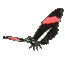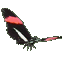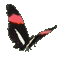Math Help - Vertical Speed - Use Tangent Line Slope of Graph - Game Tips:

- Method 1:   Speed is equal to Distance divided by Time.
The vertical speed at point P is equal to the slope of the line tangent to the curve at point P.
Use any 2 points on the tangent line to find its slope = rise/run = (change in height)/(change in time).
A previous SliderMath game, provides practice using 2 points on a line to find slope.

- Method 2: In Calculus, there is a derivative rule that calculates speed = instantaneous rate of change.
The derivative of the curve y = a(x-p)2+q is stated as dy/dx = 2a(x-p) = slope of the tangent line.
For example, the rate of change of the curve y = -4(x-3)2+6 is stated as dy/dx = 2(-4)(x-3).
At a point P on this curve, say where x=1, the speed = derivative = dy/dx = 2(-4)(1-3) = 16.

- Your Game Score is reduced by the number of butterfly hits.

- To slow the game speed repeat tap/click on the word Slider.
- To increase the game speed repeat tap/click on the word Math.
- Speed can also be adjusted with a keyboard's - and + keys.

- Refresh/Reload the web page to restart the game.